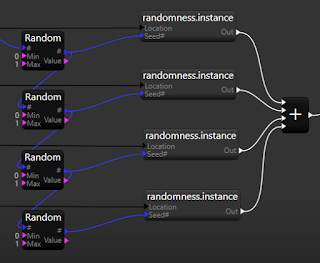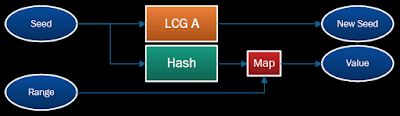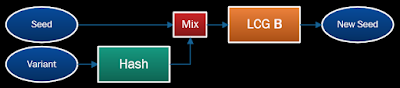## Monday 28 November 2016

### A Case Of Non-Randomness - Part 1

In preparation for a post about a randomness bug I found, let's look at some of what's going on behind the scenes in Apparance with random number generation, propagation, and manipulation.

## Premise

The random number generation in Apparance is based on two main principals:
1. Generating a repeatable sequence of random values.
2. Branching of random number sequences.
These are embodied in two operators you can build into your procedures, the "Random Value" operator (with Float and Integer variants), and the "Random Twist" operator.The two Random Value operators and the Random Twist operator
Both take a seed* value as input and produce a new seed value as an output.  The Value operators also take Range inputs and produce a Value output.  Seeds are signed (32 bit) integers and so are compatible with the normal integer connection type.
The Value operators are fairly self-explanatory but the need for the Twist operation will be explained further down.
* I've adopted the convention of using the # symbol at the end of an input or output name to indicate a seed value.

## Random Sequences

When a procedure needs some variation, it will usually be parameterised by a seed value such that it can be recreated in exactly the same way by providing the same seed, or different versions of it created by providing different seed values to each instance.Seeding a procedure.  Same seed; same output, different seed; different output.
Within the procedure, where multiple values may be needed, these can be obtained by calculating a sequence of random numbers from the given starting seed.  Each Random Value operator takes its seed from the seed output of the previous one.  This way each operation is provided with a different seed from which to generate its output value.Generating multiple values within a procedure

## Multiple Seeds

If you want to create a collection of similar, but varying, objects you would need to instance one procedure multiple times, each with a different seed.  So, how do you generate these seeds?
A simple approach would be to just use the same random generation sequence the Random Value operator uses to get each new seed (discarding the values they also generates).  However, there is a big problem with doing this; if internally your procedure uses several random values from the random sequence, the set for each instance can overlap to some extent.The problem with using a single random number generator; a rolling pattern of values
If the values in the procedure are used to drive a similar aspect of the output, e.g. the heights of several sub-elements, then you will see similarities between instances where there should be none.  This may be hidden by the exact nature of a scenario, but there are plenty of cases where this will be horribly noticeable.
Wiring up an instancing example using the procedural symbols from my Proc-jam game and using the normal random operator to generate the seeds we can investigate this effect.Investigating simple seed sequence generation problems
As the set of symbols within the instance procedure is also created using from the normal random generation operator, the symbols displayed are a window into the LCG sequence, each one shifted along by one value.Symbol sequence with sequential seeds from same generator showing the 'windowing' effect
The solution here needs some consideration of how random sequences are generated and what a seed is.

## Generation Algorithms

The sequence of values produced by a random number generator come from the random number generation algorithm it employs.  The seed is used to set up the internal state of the generator, and each time a random number is generated, the internal state changes ready to provide the next one.  The algorithm is responsible for ensuring that the output is perceived as random.  There are a huge variety of algorithms people have devised, each with different characteristics of randomness, speed, size (internal state), and cryptographic suitability (a common use case).
For procedural generation the requirements are usually just going to be around speed, and maybe storage size.  Randomness isn't normally a problem as there are many good algorithms that are fast and small.  For Apparance, one of the most basic classes of generator is all that is needed; the Linear Congruential Generator (LCG).
LCGs are particularly attractive for procedural generation use since the state can be the same size as the seed, allowing us to effectively pass the state around in the same form as we do the seeds, in fact they are treated as one-and-the-same.  This is important because for a fully deterministic procedural system this state has to be propagated around the system with all the other parameters, and this is indeed what happens in Apparance.

## Growing Seeds

A seed is the starting point for a random sequence, start with a different seed and you get a different sequence.  In fact though, what you are getting is just a different part of some larger overall sequence.  For any random number generation algorithm the sequence it generates will repeat itself eventually, but the length of this sequence is dependent on the number of possible configurations of its internal state.  The more state, the longer it can generate values without repeating itself.  For a LCG using a 32 bit integer seed there are 2^32 possible internal states and hence it would have a theoretical repeat period of over four billion values.  However, this theoretical maximum isn't guaranteed with every LCG as the mathematics used is very simple and, consequently, choosing the internal constants used is very important.  For Apparance I chose to use constants from publicly documented implementations to save the investigation and analysis time needed to derive good ones.

## Multiple Algorithms

Given that we can choose between different LCG variants, each with its own sequence of states/seeds/values, we could choose to solve our issue by using one algorithm for the inner sequence and a different one for the outer sequence.Using two different random number algorithms to avoid sequence overlap
Since the sequence of values needed by a procedure is going to be far, far smaller than the total sequence size, what we have here is multiple procedures running along different parts of the same overall sequence. For this to be successful all we needed was to change the seed to one that will not produce the next, expected, value in the sequence.  That is exactly what the other algorithm is doing, it effectively skips the needle from one part of the record to another.

## Scalability Issues

This approach has two significant downsides though:
1. This requires either more than one Random Value operator, or the operator needs to be parameterised by the algorithm used.
2. The outer procedure needs to make sure it's not using the same algorithm as the inner procedure.
The first issue here adds to the complexity of the available operators and their use.  The second introduces coupling between procedures; knowledge of the inner one is needed to use it in the outer one.  Both of these also suffer from scalability issues; it's very easy to imagine scenarios where this problem is nested more than two procedures deep, i.e. we may need more than two algorithms, or that two procedures need to be used side-by-side that are already using both algorithms.  A better solution is needed.

## Twist Variants

This 'branching' of the random streams is particularly effective, but it turns out that to solve our dependency problem it is necessary to be able to jump multiple ways from a given point in the sequence.
This is exactly what the Random Twist operator does.  It produces a new seed value using a different LCG, and also has a Variant input allowing parameterisation of this process.  This is implemented as a simple bit mangle of the variant value with the new seed value so as to change it parametrically, much like the twist operation itself, and jumping tracks again.Better use of twist operator to generate sub-seeds

## Fundamentals

It's worth summarising the random number generation processes at play here before we move on to the main story next time.
The random generation used here can be broken down into these fundamental operations:
• Generate a New Seed from a Seed
• Generate a Value from a Seed
The first is the function of the random generation algorithm (LCG in our case), and the second is actually a form of hash function.  Hash functions map values of one type or size to values of another type or size.  Commonly used to generate check-sums of large sets of data or used in encryption, they are also useful here.  The random number generation in Apparance uses a set of hash operations to map random seeds to floating point and integer values, with configurable ranges for use in the procedural generation process.
The Random Value operators are composed thus:The Random Value generation process
The Random Twist operator is composed thus:The Random Twist seed generation process
This should give you enough of a grounding in Apparances randomness operators.  Next time we'll look at the implementation of these a bit more and the quirky bug I found disrupting them.